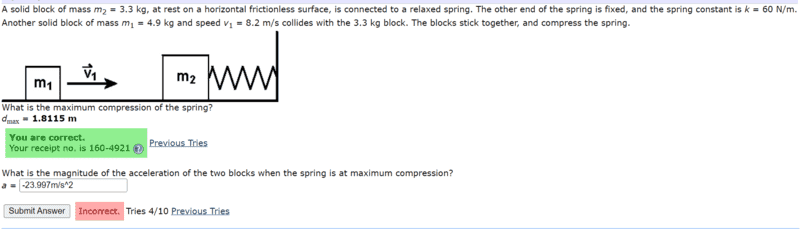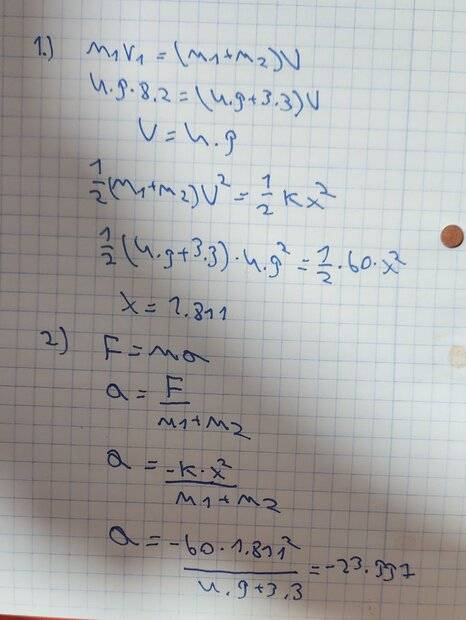# Momentum and impulse problem: A mass collides with another mass on a spring

yesmale4
Homework Statement:
A solid block of mass m2 = 3.3 kg, at rest on a horizontal frictionless surface, is connected to a relaxed spring. The other end of the spring is fixed, and the spring constant is k = 60 N/m. Another solid block of mass m1 = 4.9 kg and speed v1 = 8.2 m/s collides with the 3.3 kg block. The blocks stick together, and compress the spring.
the maximum compression of the spring is 1.8115 m.
What is the magnitude of the acceleration of the two blocks when the spring is at maximum compression?
Relevant Equations:
f=ma
m1v1=U(m1+m2)i would like to get some help and to understand why my answer is incorrect , here is how i did the first and second part.
about the first part i did it right but i don't understand what I am doing wrong in the second part i tried -k and i get -23.997 and i also try +k and i get 23.997 but they bout wrong .
i hope for some helpStaff Emeritus
Homework Helper
Gold Member
First, a couple of general comments:
- Please use units. If you had been consistent with units throughout and made dimensional checks along the way, you might have caught your error.
- Don't attach photos of your work. Write it out instead. There are several reasons for this: Your handwriting may be hard to read, your work is not quotable, etc. This is simple enough to be done with just text but for more involved things you may want to check out the LaTeX Guide.
- Your answers have too many significant digits, implying more precision than you could reasonably give. For example, your first answer of 1.8115 m has five significant digits whereas all of your inputs have only two. By all means, keep all digits in the computation, but round off the answer to a reasonable number of significant digits.

As to your issues, the problem asks for the magnitude of acceleration so it cannot be negative by definition. However, that is not your big problem as you already have understood. Instead, you may want to reexamine what expression you used for the force. By dimensional analysis of your expression
$$a = \frac{kx^2}{m_1 + m_2}$$
(corrected for the minus sign being removed in the magnitude) you would obtain length/time^2 (L/T^2) on the left hand side but (M/T^2)(L^2)/M = L^2/T^2 on the right hand side. Hence, the units on your left hand side and right hand side do not match so you should examine how this inconsistency arose.

•hutchphd
Homework Helper
Gold Member
Hm, Hooke's law is ##F=-kx## not ##F=-kx^2##...

Jackolantern
Poster has been reminded not give out answers to schoolwork questions at PF
Homework Statement:: A solid block of mass m2 = 3.3 kg, at rest on a horizontal frictionless surface, is connected to a relaxed spring. The other end of the spring is fixed, and the spring constant is k = 60 N/m. Another solid block of mass m1 = 4.9 kg and speed v1 = 8.2 m/s collides with the 3.3 kg block. The blocks stick together, and compress the spring.
the maximum compression of the spring is 1.8115 m.
What is the magnitude of the acceleration of the two blocks when the spring is at maximum compression?
Relevant Equations:: f=ma
m1v1=U(m1+m2)

View attachment 302097

i would like to get some help and to understand why my answer is incorrect , here is how i did the first and second part.
about the first part i did it right but i don't understand what I am doing wrong in the second part i tried -k and i get -23.997 and i also try +k and i get 23.997 but they bout wrong .
i hope for some help

View attachment 302098
try 13.2548 m/s^2

I guess it wouldn't be negative since as the other guys said it asks for the magnitude

yesmale4
Hm, Hooke's law is ##F=-kx## not ##F=-kx^2##...
try 13.2548 m/s^2

I guess it would be negative
ohh true I was confused thank you very much

Jackolantern
ohh true I was confused thank you very much
tell us what you get and if it says its right, please

Staff Emeritus